Courses

# Doc: Electric Field and Electric Field Lines Class 12 Notes | EduRev

## JEE: Doc: Electric Field and Electric Field Lines Class 12 Notes | EduRev

The document Doc: Electric Field and Electric Field Lines Class 12 Notes | EduRev is a part of the JEE Course Physics Class 12.
All you need of JEE at this link: JEE

Electric Field

The concept of a field was developed by Michael Faraday. An electric field intensity or simply, electric field is said to exist in the region of space around a charged object. When another charged object, (the test charge) enters this space, we say the test charge experiences an electric force, Fdue to this field.

Definition: We define the electric field due to the source charge at the location of the test charge to be the electric force on the test charge per unit charge.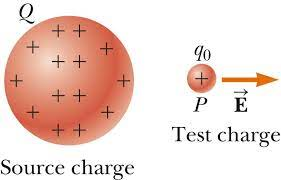In simple words, Electrostatic force per unit positive test charge is defined as Electric Field due to the source charge.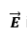is a vector quantity and its direction is same as that of force on the test charge. The unit and dimensions for Electric Field would be Newton/Coulomb  and [M1 L1 T-3I-1] respectively.

Note: The electric field is the property of its source. Presence of test charge is not necessary for the Electric field (due to source charge) to exist. It exists with or without the test charge. The test charge is used to detect and measure the Electric field due to source charge.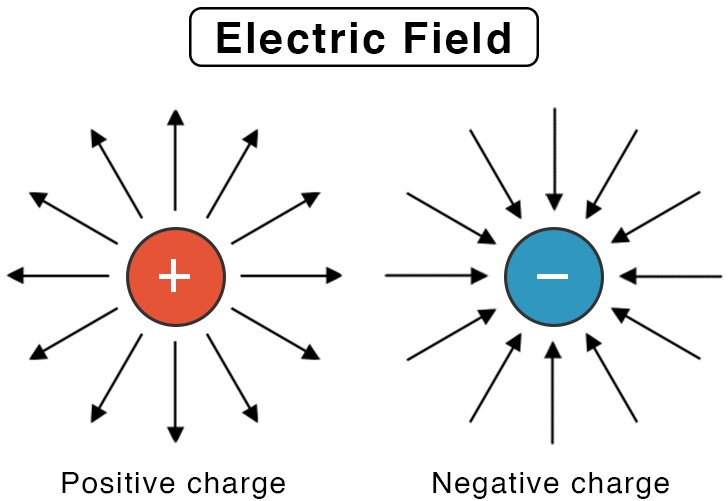Note: Observe the figure, we say that the ‘source’ charge + Q has created a region of influence around the space itself. This region of influence is visualized by defining the concept of Electric Field.

Now, if we bring any charge in this region, by Coulomb’s law, it will experience an electrostatic force. Now, the question arises, How do these charges realise that the other charge has come in its ‘territory’ or ‘region of influence’?

It happens because the other charge or ‘the test charge’ interacts with the Electric Field of the source charge and thus, electrostatic force is exerted on each other.
Conventionally, we only take the test charge to be positive. Therefore, a positive source charge would repel a ‘positive test charge’ and a negative source charge would attract a ‘positive test charge’. Thus, the electric field can be visualized in space as following: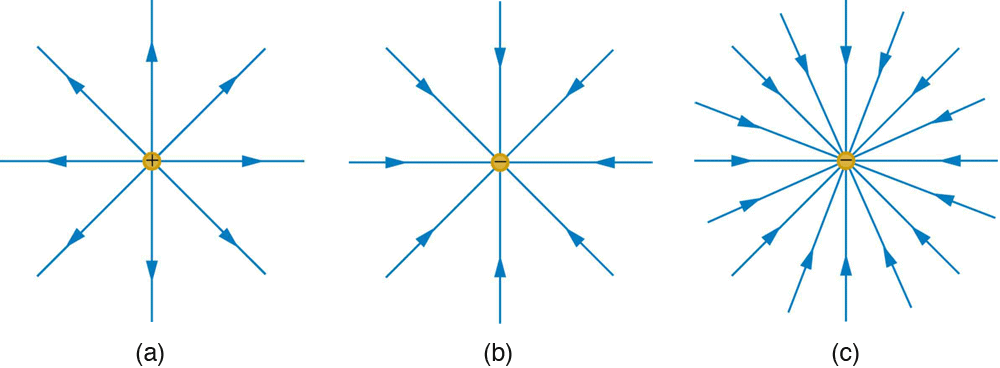The direction of electric field is radially outwards for a positive charge and is radially inwards for a negative charge as shown in the figure above based on the direction of electrostatic force on ‘positive test charge’.

There are some points to always to be kept in mind. These are
1. It is important to note that with every charged particle, there is an electric field associated which extends up to infinity.

2. No charged particle experiences force due to its own electric field.

Electric Field Strength due to Point Charge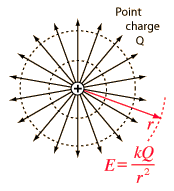As discussed earlier, if we find the electric field due to a point charge at a distance r from it. Its magnitude can be given as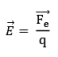By definition,
Electrostatic force on test charge +q,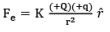Now,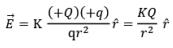Note: That if the source charge is negative i.e. –Q then we can visualise the electric field by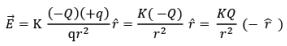This means, we can simply reverse the Electric field vector’s direction.
Thus, Electric Field due to a point charge of magnitude + Q at a distance r from it is given by the expression KQ/r2 and its direction is along the line joining the source charge and the point of consideration.

Electric Field Lines

• The concept of Electric field intensity or Electric field is visualized using Electric Field Lines.
• Electric field due to point charges we sketched the ‘region of influence’ or ‘electric field’ using lines. If the source charge is positive, then the lines are radially outwards and if the source charge is negative then the lines are radially inwards. These are not physical lines in the space, these are imaginary lines called Electric Fields lines.
• ,Any charge creates a region of influence called ‘Electric Field’ which is visualized by ‘Electric Field Lines’ and whenever other charge particle enters into this region, it experiences an ‘electrostatic force’ expressed by Coulomb’s Law.

Q. Why have we defined the concept of electric field? Is it really necessary? If eventually, we are measuring the electrostatic force, why can’t we do it directly using Coulomb’s Force?
Ans.

• When charges are stationary, the concept of electric field is convenient, but not really necessary. Electric field in electrostatics is an elegant way of characterising the electrical environment of a system of charges.
• The true physical significance of the concept of electric field, however, emerges only when we go beyond electrostatics and deal with time dependent electromagnetic phenomena.
• Suppose we consider the force between two distant charges q1, q2 in accelerated motion. The greatest speed with which a signal or information can go from one point to another is c, the speed of light. Thus, the effect of any motion of q1 on q2 cannot arise instantaneously. There will be some time delay between the effect (force on q2) and the cause (motion of q1). It is precisely here that the notion of electric field (strictly, electromagnetic field) is natural and very useful.
• The field picture is this: the accelerated motion of charge q1 produces electromagnetic waves, which then propagate with the speed c, reach q2 and cause a force on q2. The notion of field elegantly accounts for the time delay. Thus, even though electric and magnetic fields can be detected only by their effects (forces) on charges, they are regarded as physical entities, not merely mathematical constructs. They have an independent dynamics of their own, i.e., they evolve according to laws of their own. They can also transport energy. Thus concept of field is now among the central concepts in physics.

Concept of Electric Field Lines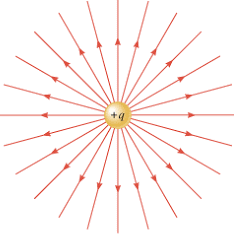Remember, this diagram, it represent electric field or the ‘region of influence’ of charge +q around the space. Now, the arrows in this diagram are called the electric field lines. Without these lines, we would not be able to visualize the concept of electric field. In this figure, each arrow indicates the electric field, i.e., the force acting on a unit positive charge, placed at the tail of that arrow. Connect the arrows pointing in one direction and the resulting figure represents a field line. We thus get many field lines, all pointing outwards from the positive point charge.

Properties of Electric Field Lines

• Field lines start from positive charges and end at negative charges. If there is a single charge, they may start or end at infinity.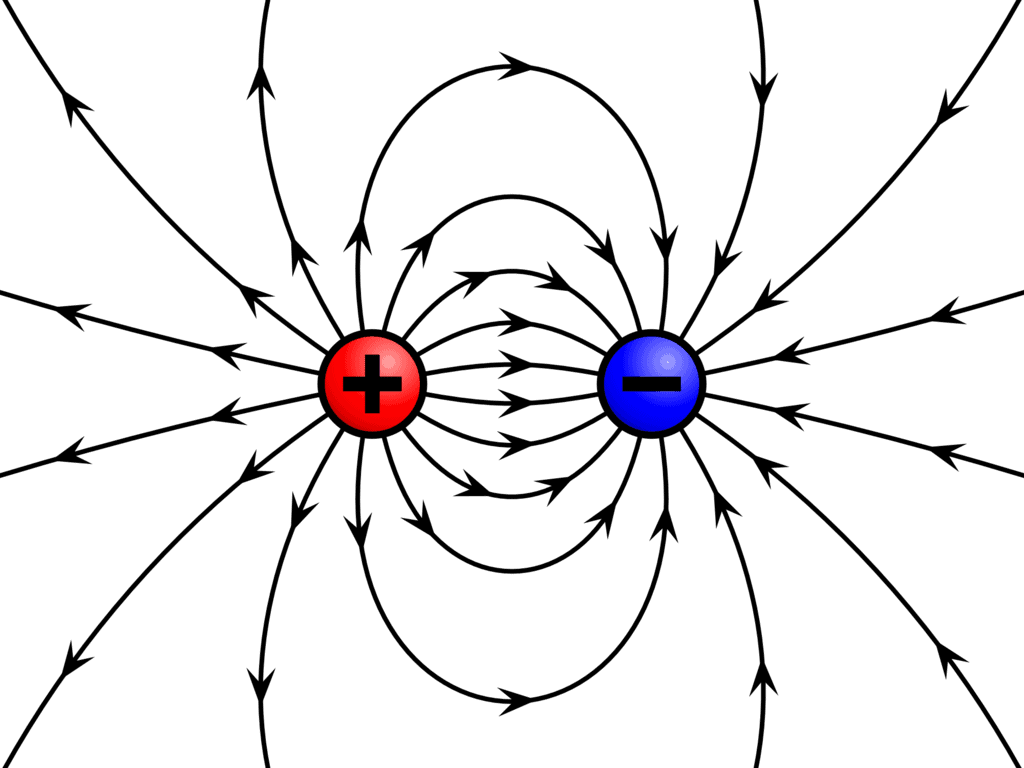• In a charge-free region, electric field lines can be taken to be continuous curves without any breaks.
• Tangent drawn to an electric field line represents the direction of electric field at that point.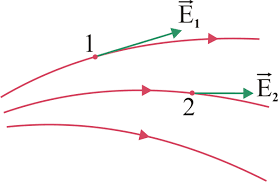• Two field lines can never cross each other. (If they did, the field at the point of intersection will not have a unique direction, which is absurd.)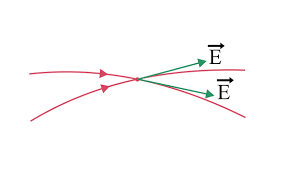• Electrostatic field lines do not form any closed loops. This follows from the conservative nature of electric field.
• The number of field lines per unit area passing through a small cross-sectional area perpendicular to the electric field is called as density of field lines.
• Relative Density of Electric Field Lines represent the magnitude of Electric Field intensity 'E', which is strong near the charge, as the density of field lines is more near the charge and the lines are closer.
• Away from the charge, the field gets weaker and the density of field lines is less, resulting in well - separated lines. Some may draw more lines but the number of lines is not important. It is the relative density of lines in different regions which is important.

Note: That electric field lines of +2Q charge are twice in number than that of +Q. So, irrespective of the number of lines in each representation, the ratio must be maintained to 2.

• Relative density of field lines is inversely proportional to the square of distance
• Mathematically, Number of lines per unit area at distance r > number of lines per unit area at distance 2r > number of lines per unit area at a distance 3r measured from S.

Relative density of field lines at distance r/Relative density of field lines at distance 2r = 4/1
Similarly,
Relative density of field lines at distance 2r/Relative density of field lines at distance 3r = 9/4

Drawing Field Lines

• Electric field lines are a way of pictorially mapping the electric field around a configuration of charges.
• An electric field line is, in general, a curve drawn in such a way that the tangent to it at each point is in the direction of the net field at that point.
• An arrow on the curve is obviously necessary to specify the direction of electric field from the two possible directions indicated by a tangent to the curve. A field line is a space curve, i.e., a curve in three dimensions.

Note: That the red dots represent positive charge and the blue dots represent the negative charge and try to verify all the properties of electric field lines listed above.

Superposition of Electric Fields

• If we are dealing with many charges (let’s assume n) then electric field at a point p is the vector sum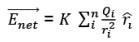• Where ri is the is the distance from the ith source charge Qi, to the point P and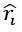is a unit vector directed from Qi toward P. If some more charge are added, more terms are added to the summation.
• However, there is no change to the terms that were already there, provided that the original charges do not move. If we know the electric fields generated by two different sets of charges separately, the electric field generated by both together is simply the vector sum of the two separate fields.
• The two fields, which each occupy three dimensional space, are superimposed on one another. Because it has this property, the electric field is said to satisfy the principle of superposition.

Graph of Electric Field Due to Binary Charge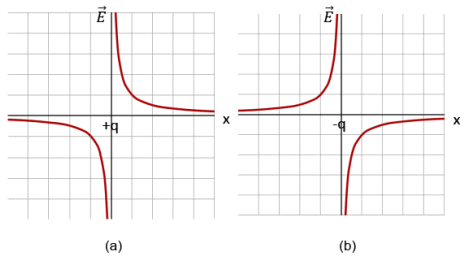(a) Electric field versus x for a positive point charge kept at the origin. Note that the electric field at positive x is positive, because it is in positive direction. At negative x it is negative, because it is in negative direction.
(b) Electric field versus x for a negative point charge kept at the origin. Note that the electric field at positive x is negative, because it is in negative direction. At negative x it is positive, because it is in positive direction.

Q. Two charged particles lie along the x-axis as shown in figure. The particle with charge q2 = +8μC is at x = 6.00 m, and the particle with charge q1 = + 2μC is at the origin. Locate the point where the resultant electric field is zero.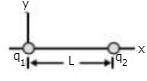Ans. Before calculating, let us physically see the location of the point where the electric field can be zero. At points other than the x -axis, say above the x-axis, both the charges will have a component of the electric field in the positive direction. This y component of the electric field does not cancel out. So the net electric field at that point will not be zero. The same statement also holds true for points which are not in xy -plane.

On the x-axis also we can see that on the points beyond x = 6 m and points on the negative x -axis, both the electric fields will be in the same direction. So the net electric field cannot be zero. At some point between the two charges, the electric field due to both of them will be in opposite direction. So the electric field will be zero at a point between x = 0 and x = 6m.
Let the x coordinate of neutral point (E = 0) be x,
Therefore,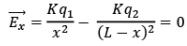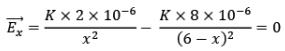By solving above equation for x, we get x = 2m.
Hence, at x = 2 between these two charged particles, there exists a point where net electric field due is zero i.e. neutral point.

The document Doc: Electric Field and Electric Field Lines Class 12 Notes | EduRev is a part of the JEE Course Physics Class 12.
All you need of JEE at this link: JEEUse Code STAYHOME200 and get INR 200 additional OFF Use Coupon Code
All Tests, Videos & Notes of JEE: JEE

### Top Courses for JEE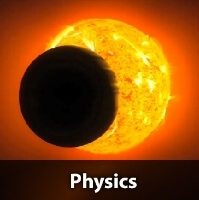## Physics Class 12

132 videos|322 docs|166 tests

### Top Courses for JEETrack your progress, build streaks, highlight & save important lessons and more!

,

,

,

,

,

,

,

,

,

,

,

,

,

,

,

,

,

,

,

,

,

;